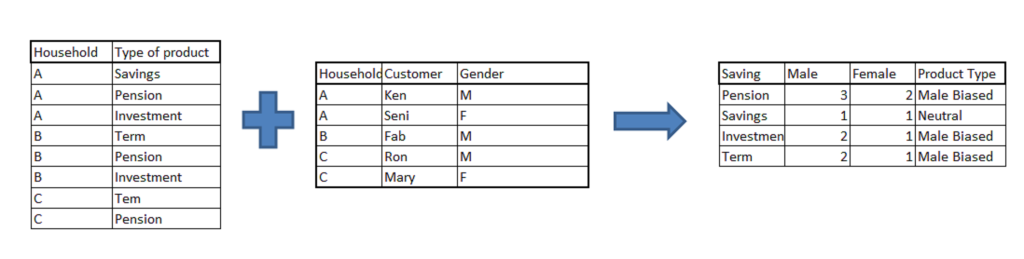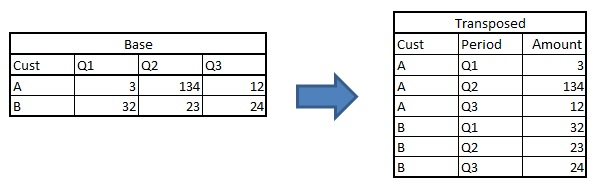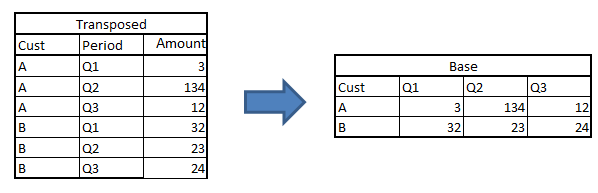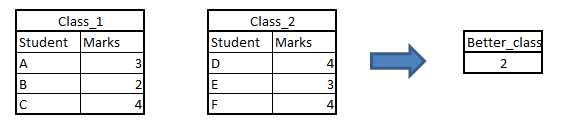# 4 tricky SAS questions commonly asked in interview

## Introduction

While working extensively on SAS-EG, I lost touch of coding in Base SAS. I had to brush up my base SAS before appearing for my first lateral interview. SAS is highly capable of data triangulation, and what distinguishes SAS from other such languages is its simplicity to code.

And if you’re looking to land your first data science role – look no further than the ‘Ace Data Science Interviews‘ course. It is a comprehensive course spanning tons of videos and resources (including a mammoth interview questions and answers guide).

1. Merging data in SAS :

Merging datasets is the most important step for an analyst. Merging data can be done through both DATA step and PROC SQL. Usually people ignore the difference in the method used by SAS in the two different steps. This is because generally there is no difference in the output created by the two routines. Lets look at the following example :Problem Statement : In this example, we have 2 datasets. First table gives the product holding for a particular household. Second table gives the gender of each customer in these households. What you need to find out is that if the product is Male biased or neutral. The Male biased product is a product bought by males more than females. You can assume that the product bought by a household belongs to each customer of that household.

Thought process: The first step of this problem is to merge the two tables. We need a Cartesian product of the two tables in this case. After getting the merged dataset, all you need to do is summarize the merged dataset and find the bias.

Code 1

`Proc sort data = PROD out =A1; by household;run;`
`Proc sort data = GENDER out =A2; by household;run;`
`Data MERGED;`
`    merge A1(in=a) A2(in=b);`
`    by household;`
`    if a AND b;`
`run;`

Code 2 :

`PROC SQL;`
`     Create table work.merged as`
`           select t1.household,  t1.type,t2.gender`
`           from prod as t1, gender as t2`
`           where t1.household = t2.household;`
`quit; `

Will both the codes give the same result?

The answer is NO. As you might have noticed, the two tables have many-to-many mapping. For getting a cartesian product, we can only use PROC SQL. Apart from many-to-many tables, all the results of merging using the two steps will be exactly same.

Why do we use DATA – MERGE step at all?

DATA-MERGE step is much faster compared to PROC SQL. For big data sets except one having many-to-many mapping, always use DATA- MERGE.

2. Transpose data-sets :

When working on transactions data, we frequently transpose datasets to analyze data. There are two kinds of transposition. First, transposing from wide structure to narrow structure. Consider the following example :Following are the two methods to do this kind of transposition :

a. DATA STEP :

`data transposed;set base;`
`        array Qtr{3} Q:;`
`        do i = 1 to 3;Period = cat('Qtr',i);Amount = Qtr{i} ;output;end;`
`        drop Q1:Q3;`
`        if Amount ne .;`
`run; `

b. PROC TRANSPOSE :

```proc transpose data = base out = transposed
(rename=(Col1=Amount) where=(Amount ne .)) name=Period;```
`by cust; run; `

In this kind of transposition, both the methods are equally good. PROC TRANSPOSE however takes lesser time because it uses indexing to transpose.

Second, narrow to wide structure. Consider an opposite of the last example.For this kind of transposition, data step becomes very long and time consuming. Following is a much shorter way to do the same task,

`Proc transpose data=transposed out=base (drop=_name_) prefix Q;`
`       by cust;`
`       id period;`
`       var amount;`
`run; `

3. Passing values from one routine to other:

Imagine a scenario, we want to compare the total marks scored by two classes. Finally the output should be simply the name of the class with the higher score. The score of the two datasets is stored in two separate tables.

There are two methods of doing this question. First, append the two tables and sum the total marks for each or the classes. But imagine if the number of students were too large, we will just multiply the operation time by appending the two tables. Hence, we need a method to pass the value from one table to another. Try the following code:`DATA _null_;set class_1;`
`       total + marks;`
`       call symputx ('class1_tot',total);`
`run;`
`DATA _null_;set class_2;`
`       total + marks;`
`       call symputx ('class2_tot',total);`
`run;`
`DATA results;`
`       if &class1_tot > &class2_tot then better_class = 1;`
`       else if &class1_tot > &class2_tot then better_class = 2;`
`       else better_class = 0;`
`run; `

Funtion symputx creates a macro variable which can be passed between various routines and thus gives us an opportunity to link data-sets.

4. Using where and if :

“Where” and “if” are both used for sub-setting. Most of the times where and if can be used interchangeably in data step for sub-setting. But, when sub-setting is done on a newly created variable, only if statement can be used. For instance, consider the following two programs,

Code 1 :                                                                                  Code 2 :

`data a;set b;                            data a;set b;`
`      z= x+y;                                  z= x+y;`
`      if z < 10;                               where z < 10;`
`run;                                     run; `

Code 2 will give an error in this case, because where cannot be used for sub-setting data based on a newly created variable.

End Notes :

These codes come directly from my cheat chit. What is especial about these 4 codes, that in aggregate they give me a quick glance to almost all the statement and options used in SAS. If you were able to solve all the questions covered in this article, we think you are up for the next level. You can read the second part of this article here ( https://www.analyticsvidhya.com/blog/2014/04/tricky-base-sas-interview-questions-part-ii/ ) . The second part of the article will have tougher and lengthier questions as compared to those covered in this article.

Have you faced any other SAS problem in analytics interview? Are you facing any specific problem with SAS codes?  Do you think this provides a solution to any problem you face? Do you think there are other methods to solve the problems discussed in a more optimized way? Do let us know your thoughts in the comments below.

## If you like what you just read & want to continue your analytics learning, subscribe to our emails or like our facebook page.

You can also read this article on Analytics Vidhya's Android APP•Priya Varshney says:

1. Merging data in SAS :
Data A;
Input household \$ type_of_product \$13.;
Datalines;
A savings
A pension
A investment
B term
B pension
B investment
C term
C pension
;

run;
proc print;
run;
Data B;
input household \$ Customer \$ gender \$;
datalines;
A Ken M
A Seni F
B Fab M
C Ron M
C Mary F
;
run;
Proc sort data = a out =A1; by household;run;
Proc sort data = b out =A2; by household;run;
Data MERGED;
merge A1(in=a) A2(in=b);
by household;
if a AND b;
run;

proc print data=merged;
run;
PROC SQL;
Create table work.merged as
select t1.household, t1.type,t2.gender
from a as t1, b as t2
where t1.household = t2.household;
quit;
proc sort data=merged out=f;
by type_of_product;
run;

data final;
retain f 0;
retain m 0;
set f;
by type_of_product;
if gender=”F” then f=f+1;
if gender=”M” then m=m+1;
if last.type_of_product then do;
if f>m then product_type=”Female based”;
else
if m>f then product_type=”Male based”;
else
product_type=”Neutral”;
output;
f=0;
m=0;
end;
drop household customer gender f m ;
run;

proc print data=final;
run;

•Priya Varshney says:

Data A;
Input household \$ type_of_product \$13.;
Datalines;
A savings
A pension
A investment
B term
B pension
B investment
C term
C pension
;

run;
proc print;
run;
Data B;
input household \$ Customer \$ gender \$;
datalines;
A Ken M
A Seni F
B Fab M
C Ron M
C Mary F
;
run;
Proc sort data = a out =A1; by household;run;
Proc sort data = b out =A2; by household;run;
Data MERGED;
merge A1(in=a) A2(in=b);
by household;
if a AND b;
run;

proc print data=merged;
run;
PROC SQL;
Create table work.merged as
select t1.household, t1.type,t2.gender
from a as t1, b as t2
where t1.household = t2.household;
quit;
proc sort data=merged out=f;
by type_of_product;
run;

data final;
retain f 0;
retain m 0;
set f;
by type_of_product;
if gender=”F” then f=f+1;
if gender=”M” then m=m+1;
if last.type_of_product then do;
if f>m then product_type=”Female based”;
else
if m>f then product_type=”Male based”;
else
product_type=”Neutral”;
output;
f=0;
m=0;
end;
drop household customer gender f m ;
run;

proc print data=final;
run;

•Anders Sköllermo says:

Hi! COMMENT to the answer to “4. Using where and if : ”

Suppose SAS-table B (WORK.B) contains a variable B, then the solution in Code 2 will work:
data a;set b; z= x+y; /* The old value of Z, which was < 10, will be replaces. */ where z < 10; run;

The WHERE-clause should (in my opinion) immediately follow the SET-statement.

Basic questions: What data do You have ? What rules do you have? What results do you want ?
THEN we can start to discuss what is CORRECT and what is (perhaps) "a little less correct"
(British English understatement).
/ Br Anders

•sandy says:

Simple diff b/w IF and WHERE CLAUSE.
IF works on pdv, where as WHERE works on source and applies conditions so new cant be created in WHERE.
so there In code2 shows error.

•Anders Sköllermo says:

Hi! Type error. My note should read: “Suppose SAS-table B (WORK.B) contains a variable Z, …”
(The drawback of writing the answers late at night..).

In other words: If you have a variable Z and also create a variable Z, then the result depends strongly
on the use of WERE (the old Z value) or IF (the newly calculated Z value).

The programmers show give some thoughts to data structures, what variables are created where, what results are wanted.
/ Br Anders

•Anders Sköllermo says:

Case 4: Bottom Line: Case 2 with WHERE may work “fine”, without any errors at all.

•Tavish says:

Anders,
Thanks for bringing this up. You are right in saying that in case “z” is already a variable of the set data-set, the code won’t show error. But the objective of bringing this up was that , as sandy quoted, “IF works on pdv, where as WHERE works on source and applies conditions” . But that was a sharp catch for sure.

Tavish

•bhanu says:

Hi Tavish,

I do know about base sas and macros but i want to know more about banking projects how it goes on the daily basis.I want to know about the work of the analyst in a banking domain from the scratch.Can u please help me in guiding me as u r also from the same domain.I really appreciate your efforts u r investing for the young professionals.

Thanks in Anticipation
Bhanu

•Shivi says:

Following on email

•Anders Sköllermo says:

Hi! The real meaning of the WHERE-statement:
The WHERE statement is (approximately) a WHERE data set option:
data a;
set b (where=(z < 10));
Z= x+y;
run;

The WHERE statement cannot be executed conditionally. That is, you cannot use it as part of an IF-THEN statement. (according to SAS9.3 manuals).

data a;
set b;
where=z < 10;
Z= x+y;
run;

Please note that the following is OK (but I would NEVER write like that)
data a;
where=z < 10; /* WHERE on SET WORK.B – not on WORK.A ! */
set b;
Z= x+y;
run;

The following is OK and works as it should – BUT DO NOT USE IT !!
data work.a;
if x=1 then do;
where=z < 10;
end; /* The WHERE-part is a declaration and will always be used! */
set work.b;
z= x+y;
run;

My way of writing SAS programs: Learn the actual syntax and functionality of all the parts that you use.
Write very clear SAS-programs with a lot of documentation.

•Anders Sköllermo says:

Use WHERE-data set option and IF statement together!

It can be handy to use the WHERE data set option (perhaps written as the WHERE statement) to restrict what observation are used in the data step AND one or several IF statements do do further calculations and selections on these observations, using the newly calculated variables.

•DaveG says:

hi,

good set of four questions.

the proc transpose vs the data step: These programs do the same thing but they are *not* equivalent or equally useful.
Why?

The data step as programmed has to have 3 occurences per set, exactly three.
The Proc transpose works for any number of occurences (and creates any number of variables when ging from thin to wide).

In practical use this is important and I would not accept the assertion these methods are the same if I heard that in an interview.

•Tavish Srivastava says:

Dave,
You made a really good point here. As is, data step and proc transpose do the same job strictly for the data set mentioned in the article. However, if the number of variable increase you need to change the array length.

But, in case you want to make the array length variable, it is indeed possible. Here is how it can be done,
1.Replace 3 by “*” in array definition.
2. Make a variable which will take the length of the array.
3. Use the defined variable in the Do loop.

Do let me know in case you still disagree.

Tavish

•DaveG says:

BTW it is easy to do example 3 with one datastep and no macro variables. 🙂

•Tavish Srivastava says:

Dave,
It will be great for us and our viewers if you illustrate how it can be done in one data-step. I have no doubts that you know such a method 😉

Tavish

•priya says:

alternative solution for case1 using proc sql:

data one;
infile datalines;
input household \$ product_type \$10.;
datalines;
A Savings
A Pension
A Investment
B Term
B Pension
B Investment
C Term
C Pension
;

data two;
infile datalines;
input household \$ Customer \$ Gender \$;
datalines;
A Ken M
A Seni F
B Fab M
B Ron M
C Mary F
;

proc sort data=one;
by household;
run;
proc sort data=two;
by household;
run;

PROC SQL;

Create table work.merged as
select t1.household, t1.product_type,t2.gender
from one as t1, two as t2
where t1.household = t2.household;

create table male(drop=gender)
as select distinct product_type as saving,gender,count(gender) as Male
from merged
group by saving,gender
having gender=’M’;

create table female(drop=gender)
as select distinct product_type as saving,gender,count(gender) as female
from merged
group by saving,gender
having gender=’F’;

create table joined as select m.saving,male,female,
case
when male>female then ‘Male-based’
when female>male then ‘Female-based’
else ‘Neutral’
end as biased
from male m,female f
where m.saving=f.saving
order by saving;
quit;

•Aparna Konda says:

What is the major difference between Datalines and Cards in SAS programming?

•Shreesh says:

Before datalines come in sas cards are used, its a version up-gradation.
it is also note hat cards works on infile also.

•Kunal Jain says:

Thanks Shreesh

•Anu says:

In short: No difference.

In detail: Before the invention of terminals/keyboards, programs (software) and data all were prepared in cards with special punching machines that make holes in the cards to represent numbers and characters. In those years SAS developers used the statement cards to refer data, indirectly meaning “beyond this card, data are provided”. In fact they should have used the term “datacards” instead of cards.

Most of the programs are written once but used repeatedly. Data are varying for each use. So we prepare the programs, of course, in cards. After verifying it works correct we record the program in Tapes or disks permanently. Second time on wards we call the program from tape or disks and supply the data via cards. To each job, there will a few cards at the beginning of the card stack that will have job control lines giving information about the storage location and name of the program. Then data follow after a card that carries the message “cards”.

Other than this reason, there is absolutely no difference between cards and datalines.

•Anders says:

In 1976 when SAS was (officially) released, data were read from records 80 bytes broad.
Often the data were included, so you first had the Data step, which ended with CARDS; statement. After that came the data rows. The end of the data cards / data rows was signalled using RUN;

DATALINES means exactly the same as CARDS (as far as I know).

Often it is better to store the data in a separate file, which you refer to using the INFILE statement. Then specify how to read the data using the INPUT statement. CARDS or DATLINES is not used.
/ BAr Anders

•Kunal Jain says:

Thanks Anders for answering the question.

Regards,
Kunal

•Anders says:

Please note that INFILE CARDS; is the default. This means that after the Cards; or Datalines; statement, there will be datalines. (CARDS on the INFILE statement is an option for INFILE).
/ Br Anders

•Amanpreet says:

Hi Kunal,

Nice questions above.
Similarly can u share an example for nodup and nodupkey.

This is confusing me.

Thanks aman

•Deep says:

Can we use proc sql to draw the final table instead of retain….( specially the count of gender male / female)

•Arun says:

Hi I’m Arun from Bangalore, I work in reputed company as a SLM co-ordinator. I want to switch to a technology field and when i was looking found Analysts and SAS to be pretty interesting. I have around 9 years of industry experience in Service management. And my salary is around 10 lakhs per anum . Wanted to know if i switch my carrier now and do the relevant courses necessary for analysts and SAS will this be a wise idea. And in terms of finance as well will it be a wise move.

•rsakumar says:

hai sir very good perform sas better understanding

•pratik singh says:

As far as I have studied …Comparing proc sql and datastep merge:
When dataset is small both take appox same resource(for one to one and many to one)..but as the size increases proc sql starts getting better.Whether it be in terms of I/O operations time or space.(If the dataset is not already sorted) .I read in one of the SAS User Group Paper

•Anders Sköllermo says:

3. Passing values from one routine to other:

The following code:
DATA _null_;set class_1;
total + marks;
call symputx (‘class1_tot’,total);
run;

Please note that this means that CALL SYMPUTX is called once in EVERY loop of the Data step.
SO this works fine on small sets of data, but is Expensive on large sets of data. Suggested solution:
DATA Sum_class_1;
set class_1 end= endclass1;
total + marks;
if endclass1 then call symputx (‘class1_tot’,total);
run;
I have not made any test run, but the solution is basically OK).
/Best Regards Anders
DATA _null_;set class_2;